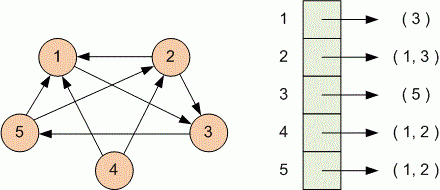Problems

# Count sources

Time limit 1 second
Memory limit 128 MiB

The directed graph is given with the adjacency list. The vertex of directed graph is called a source if no edge comes into it. Count the number of sources in the graph.

## Input data

First line contains the number of vertices n (1n100). The next i-th line contains the number of edges adjacent to the i-th vertex, and the vertex numbers where these edges go in increasing order.

## Output data

Print the number of sources in the graph.#### Example

Graph contains 1 source - the vertex number 4.

## Examples

Input example #1
5
1 3
2 1 3
1 5
2 1 2
2 1 2

Output example #1
1

Author Michael Medvedev